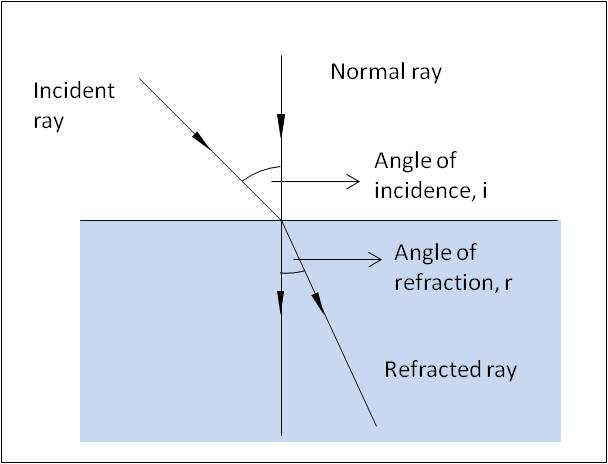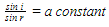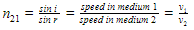# Refraction

## Introduction

Refraction is the change of direction of wave that occurs when its speed changes. Refraction is a phenomenon that is exhibited by wave when it travels from one medium to another, for example, when light travels from air to glass or vice versa. Refraction can be also be seen when water waves move from deeper into shallower water.

A. Refraction

Consider figure 1 that shows the refraction of light when it travels from air to glass. The normal ray is perpendicular to the barrier. The incident ray form an angle with the normal, called as angle of incidence (i). This light ray bends when it moves to glass due to change in speed. The refracted ray is represented and it forms an angle with the normal, called as the angle of refraction (r).Figure 1: Refraction of Light

B. Refractive Index

A straightforward relationship is found between the angle of incidence and angle of refraction by various experiments. This relationship is called Snell’s law and is mathematically represented by the equation shown below.This constant is called as the refractive index and letter n is used to represent it. It is common to use subscripts to indicate the medium in which the wave starts and finishes. When the wave moves from medium 1 to medium 2, Snell’s law is written as,Refractive index of various substances is shown in table below.C. Wave Speed and Refraction

Refraction occurs because of the change in speed of the wave.  The amount of refraction depends on the amount of speed change. The refractive index is a measure of how much a ray bends when moving from one medium to another. Refractive index is also equal to the ratio of the speeds in the two media.For rays entering medium 1 from medium 2, the refractive index is written mathematically as,D. Total Internal Reflection

When the light ray travels from an optically dense medium to a less dense medium, the rays are refracted away from the normal because the speed of light increases. This can be experimentally investigated by shining the light through a semi-circular block and taking the measurements of angle inside and outside the block from the flat side of the block.

As the angle of incidence inside the glass block gradually increases, the angle of refraction becomes larger and larger, always being greater than the angle inside the block. If the internal angle is increased beyond a value, the ray no longer leaves the glass. It reflects inside, following the law of reflection. In this case, there is no refraction –the ray has been totally internally reflected. This phenomena is called the total internal reflection. It is illustrated in Figure 2.Figure 2: Total Internal Reflection

E. Critical Angle

Critical angle is the angle of incidence when the refracted ray leaves at 90° to the normal. Critical angle is illustrated in Figure 3. In the example, refracted light leaves at 90° to the normal when the angle of incidence is Өc. This value is the critical angle of refraction. Critical angle is the largest angle of incidence for which refraction can still occur. For any angle of incidence greater than the critical angle, light will undergo total internal reflection.Figure 3: Critical Angle

F. Optical Fibres

Optical fibres are communication cables used in medical instruments called endoscope, telephone, Internet and cable TV. The use of optical fibres in communications has improved the transmission of data giving us high speed internet access. Figure 4 illustrates how an optical fibre transport light wave.Figure 4: Optical Fibre

As illustrated in the above figure, an optical fibre has a fine glass core and it is surrounded by a cladding of glass with a lower refractive index than the core. This means that light shone into the core at an angle greater than the critical angle (Өc) will total internally reflect at the boundary between the core and the cladding. The light then travels down the fibre through a series of reflections before exiting at the other end.

G. Summary

• Refraction is the change of direction of wave that occurs when its speed changes.
• Angle formed by the incident ray with the normal is called as angle of incidence (i).
• Angle formed by the refracted ray with the normal is called as angle of refraction (r).
• Refractive index is the ratio of sin i and sin r.
• When total internal reflection happens, the ray no longer leaves the glass. It reflects inside, following the law of reflection. In this case, there is no refraction –the ray has been totally internally reflected.
• Critical angle is the angle of incidence when the refracted ray leaves at 90° to the normal.
• In fibre optical cable, the light enters at critical angle and travels through the fibre by a series of reflections before exiting at the other end.